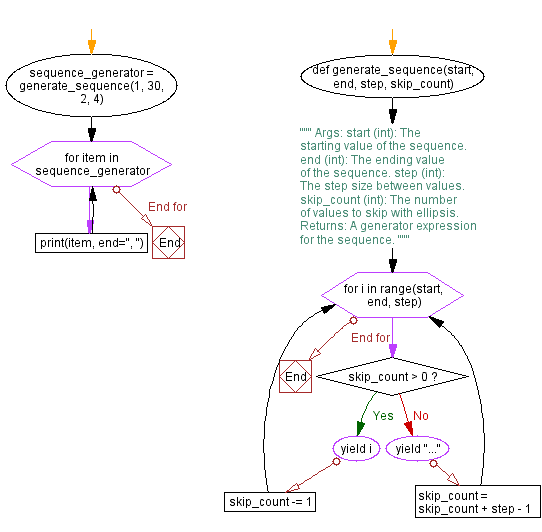﻿ Generating sequences with ellipsis in Python

# Generating sequences with ellipsis in Python

## Python ellipsis (...) Data Type: Exercise-7 with Solution

Write a Python program to create a generator expression that generates a sequence of numbers with ellipsis representing skipped values.

Sample Solution:

Code:

``````def generate_sequence(start, end, step, skip_count):
"""
Args:
start (int): The starting value of the sequence.
end (int): The ending value of the sequence.
step (int): The step size between values.
skip_count (int): The number of values to skip with ellipsis.

Returns:
A generator expression for the sequence.
"""
for i in range(start, end, step):
if skip_count > 0:
yield i
skip_count -= 1
else:
yield "..."
skip_count = skip_count + step - 1

sequence_generator = generate_sequence(1, 30, 2, 4)

for item in sequence_generator:
print(item, end=", ")
``````

Output:

```1, 3, 5, 7, ..., 11, ..., 15, ..., 19, ..., 23, ..., 27, ...,
```

In the exercise above, the "generate_sequence()" function takes parameters for the starting value ('start'), ending value ('end'), step size ('step'), and the number of values to skip with 'ellipsis' (skip_count). It uses a generator expression to yield values from 'start' to 'end' with the specified step size. When it reaches 'skip_count', it yields 'ellipsis (...)' to represent skipped values.

Flowchart:What is the difficulty level of this exercise?

Test your Programming skills with w3resource's quiz.

﻿

## Python: Tips of the Day

Summing a sequence of numbers (calculating the sum of zero to ten with skips):

```>>> l = range(0,10,2)
>>> sum(l)
20
```

We are closing our Disqus commenting system for some maintenanace issues. You may write to us at reach[at]yahoo[dot]com or visit us at Facebook